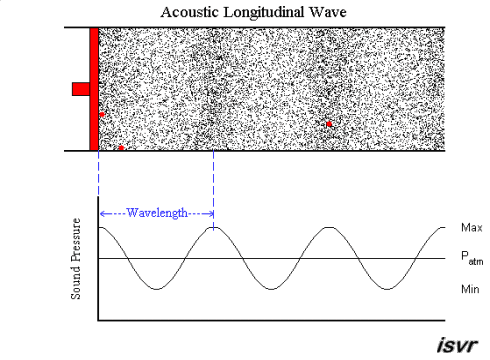BUY THE YEAR 9 PHYSICS WORKBOOK

## Representing Sound Waves Graphically

• Sound waves can be represented graphically, as shown below.• The peaks on a graph of a sound wave correspond to compressions (maximum pressure).
The troughs on a graph of a sound wave correspond to rarefactions (minimum pressure).
• The size of the peaks represents the wave’s amplitude and corresponds to how much the particles are vibrating.
• The distance between two peaks represents the wave’s wavelength and corresponds to how far the wave travels during one cycle.
• Frequency (not shown on the graph) corresponds to the number of wave cycles per second. It is measured in hertz (Hz).
• Remember, a graph of a wave is not an actual description of how particles in the wave move. Even though they look like transverse wave motion, sound waves are longitudinal waves and do not look like this when they travel through matter. See the diagram below for a comparison of particle movement in a sound wave and the corresponding graph.(Image: © Institute of Sound and Vibration Research, University of Southampton)